Courses

# Types of Motion and Explanation of Simple Harmonic Motion Class 11 Notes | EduRev

## Class 11 : Types of Motion and Explanation of Simple Harmonic Motion Class 11 Notes | EduRev

The document Types of Motion and Explanation of Simple Harmonic Motion Class 11 Notes | EduRev is a part of the Class 11 Course Physics Class 11.
All you need of Class 11 at this link: Class 11

Oscillations:

Periodic Motion:

A motion which repeats itself identically after a fixed interval of time is called periodic motion. e.g., orbital motion of the earth around the sun, a motion of arms of a clock, a motion of a simple pendulum etc.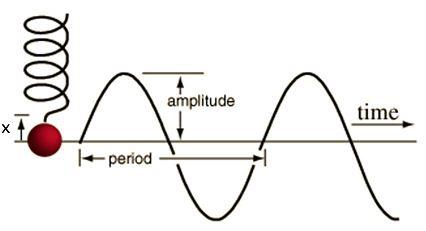Fig: Periodic motion

Oscillatory Motion:

A periodic motion taking place to and fro or back and forth about a fixed point is called oscillatory motion, e.g., motion of a simple pendulum, motion of a loaded spring etc.

Note- Every oscillatory motion is periodic motion but every periodic motion is not oscillatory motion.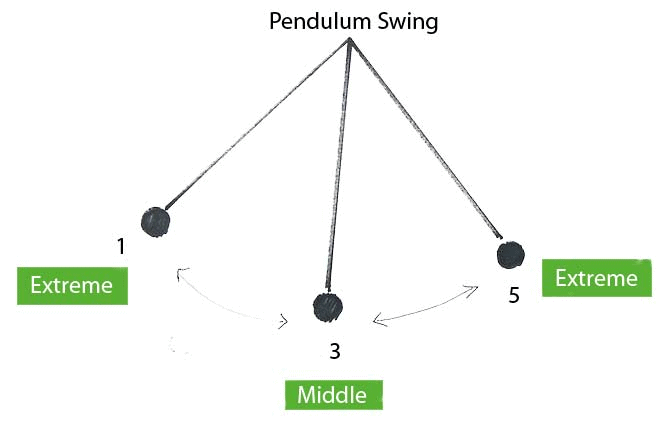Fig: Swinging pendulum

Harmonic Oscillation:

The oscillation which can be expressed in terms of single harmonic function, i.e., sine or cosine function, is called harmonic oscillation.

Simple Harmonic Motion:

A harmonic oscillation of constant amplitude and of single frequency under a restoring force whose magnitude is proportional to the displacement and always acts towards mean Position is called Simple Harmonic Motion (SHM).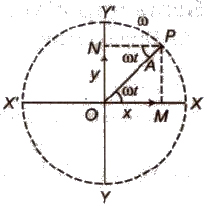A simple harmonic oscillation can be expressed as

y = a sin ωt

or y = a cos ωt

Where a = amplitude of oscillation.

Non-harmonic Oscillation:

A non-harmonic oscillation is a combination of two or more than two harmonic oscillations.

It can be expressed as y = a sin ωt + b sin 2ωt

Some Terms Related to SHM:

(i) Time Period: Time taken by the body to complete one oscillation is known as time period. It is denoted by T.

(ii) Frequency: The number of oscillations completed by the body in one second is called frequency. It is denoted by v.

Its SI unit is ‘hertz’ or ‘second-1‘.

Frequency = 1 / Time period

(iii) Angular Frequency: The product of frequency with factor 2π is called angular frequency. It is denoted by ω.

Angular frequency (ω) = 2πv

Its SI unit is ‘hertz’ or ‘second-1‘.

(iv) Displacement: A physical quantity which changes uniformly with time in a periodic motion is called displacement. It is denoted by y.

(v) Amplitude: The maximum displacement in any direction from mean position is called amplitude. It is denoted by a.

(vi) Phase: A physical quantity which express the position and direction of motion of an oscillating particle, is called phase. It is denoted by φ.

Simple harmonic motion is defined as the projection of a uniform circular motion on any diameter of a circle of reference.

Some Important Formula of SHM:

(i) Displacement in SHM at any instant is given by

y = a sin ωt

or y = a cos ωt

where a = amplitude and

ω = angular frequency.

(ii) Velocity of a particle executing SHM at any instant is given by

v = ω √(a2 – y2)

At mean position y = 0 and v is maximum

vmax = aω

At extreme position y = a and v is zero.

(iii) Acceleration of a particle executing SHM at any instant is given by

A or α = – ω2 y

Negative sign indicates that the direction of acceleration is opposite to the direction in which displacement increases, i.e., towards mean position.

At mean position y = 0 and acceleration is also zero.

At extreme position y = a and acceleration is maximum

Amax = – aω2

(iv) Time period in SHM is given by

T = 2π √Displacement / Acceleration

Graphical Representation:

(i) Displacement – Time Graph: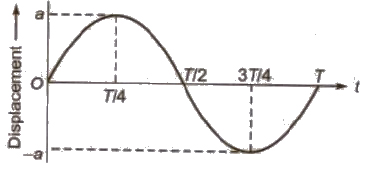(ii) Velocity – Time Graph: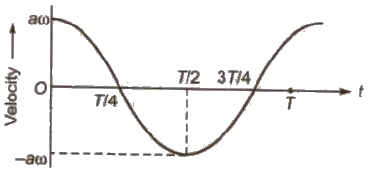(iii) Acceleration – Time Graph: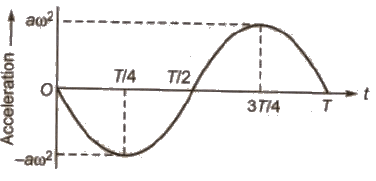Note The acceleration is maximum at a place where the velocity is minimum and vice – versa.

For a particle executing SHM, the phase difference between

(i) Instantaneous displacement and instantaneous velocity

(ii) Instantaneous velocity and instantaneous acceleration

(iii) Instantaneous acceleration and instantaneous displacement

The graph between velocity and displacement for a particle executing SHM is elliptical.

Force in SHM:

We know that, the acceleration of body in SHM is α = -ω2 x

Applying the equation of motion F = ma,

We have, F = – mω2 x = -kx

Where, ω = √k / m and k = mω2 is a constant and sometimes it is called the elastic constant.

In SHM, the force is proportional and opposite to the displacement.

Energy in SHM:

The kinetic energy of the particle is K = 1 / 2 mω2 (A2 – x2)

From this expression we can see that, the kinetic energy is maximum at the centre (x = 0) and zero at the extremes of oscillation (x ± A).

The potential energy of the particle is U = 1 / 2 mω2 x2

From this expression we can see that, the potential energy has a minimum value at the centre (x = 0) and increases as the particle approaches either extreme of the oscillation (x ± A).

Total energy can be obtained by adding potential and kinetic energies. Therefore,

E = K + U

= 1 / 2 mω2 (A2 – x2) + 1 / 2 mω2 x2

= 1 / 2 mω2 A2

where A = amplitude

m = mass of particle executing SHM.

ω = angular frequency and

v = frequency

Changes of kinetic and potential energies during oscillations.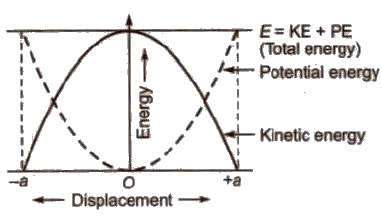The frequency of kinetic energy or potential energy of a particle executing SHM is double than that of the frequency in SHM.

The frequency of total energy of particles executing SHM is zero as total energy in SHM remains constant at all positions.

When a particle of mass m executes SHM with a constant angular frequency (I), then time period of oscillation

T = 2π √Inertia factor / Spring factor

In general, inertia factor = m, (mass of the particle)

Spring factor = k (force constant)

How the different physical quantities (e.g., displacement, velocity, acceleration, kinetic energy etc) vary with time or displacement are listed ahead in tabular form.

 S.No. Name of the equation Expression of the equation Remarks 1. Displacement-time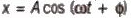x varies between + A and - A 2. Velocity-time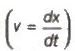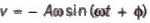v varies between + Aω and - A ω 3. Acceleration-time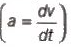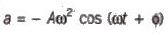a varies between + Aω2 and - Aω2 4. Kinetic energy-time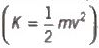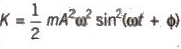K varies between and5. Potential energy-time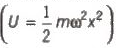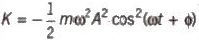U varies between and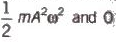6. Total energy-time(E = K + U)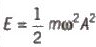E is constant 7. Velocity-displacement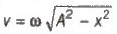v = 0 at x = ±4 and at x = 0 , v = ± Aω 8. Acceleration-displacement a = - ω2X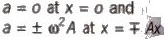9. Kinetic energy-displacement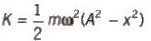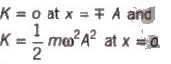10. Potential energy-d displacement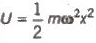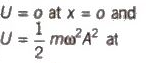11. Total energy-d displacement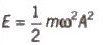x = ± A E is constant

Oscillations of Liquid in a U – tube:

If a liquid is filled up to height h in both limbs of a U-tube and now liquid is depressed upto a small distance y in one limb and then released, then liquid column in U-tube start executing SHM.

The time period of oscillation is given by

T = 2π √h / g

Oscillations of a floating cylinder in liquid is given by

T = 2π √l / g

where I = length of the cylinder submerged in liquid in equilibrium.

When a spring is compressed or stretched through a small distance y from mean position, a restoring force acts on it.

Restoring force (F) = – ky

where k = force constant of spring.

If a mass m is suspended from a spring then in equilibrium,

mg = kl

This is also called Hooke’s law.

Time period of a loaded spring is given by:

T = 2π √m / k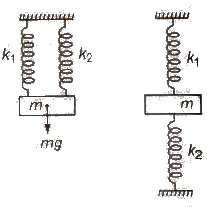When two springs of force constants k1 and k2 are connected in parallel to mass m as shown in figure, then

(i) Effective force constant of the spring combination

k = k1 + k2

(ii) Time period T = 2π √m / (k1 + k2)

When two springs of force constant k1 and k2 are connected in series to mass m as shown in figure, then

(i) Effective force constant of the spring combination

1 / k = 1 / k1 + 1 / k2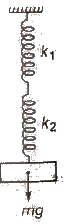(ii) Time period T = 2π √m(k1 + k2) / k1k2

Free Oscillations:

When a body which can oscillate about its mean position is displaced from mean position and then released, it oscillates about its mean position. These oscillations are called free oscillations and the frequency of oscillations is called natural frequency.

Offer running on EduRev: Apply code STAYHOME200 to get INR 200 off on our premium plan EduRev Infinity!

,

,

,

,

,

,

,

,

,

,

,

,

,

,

,

,

,

,

,

,

,

;